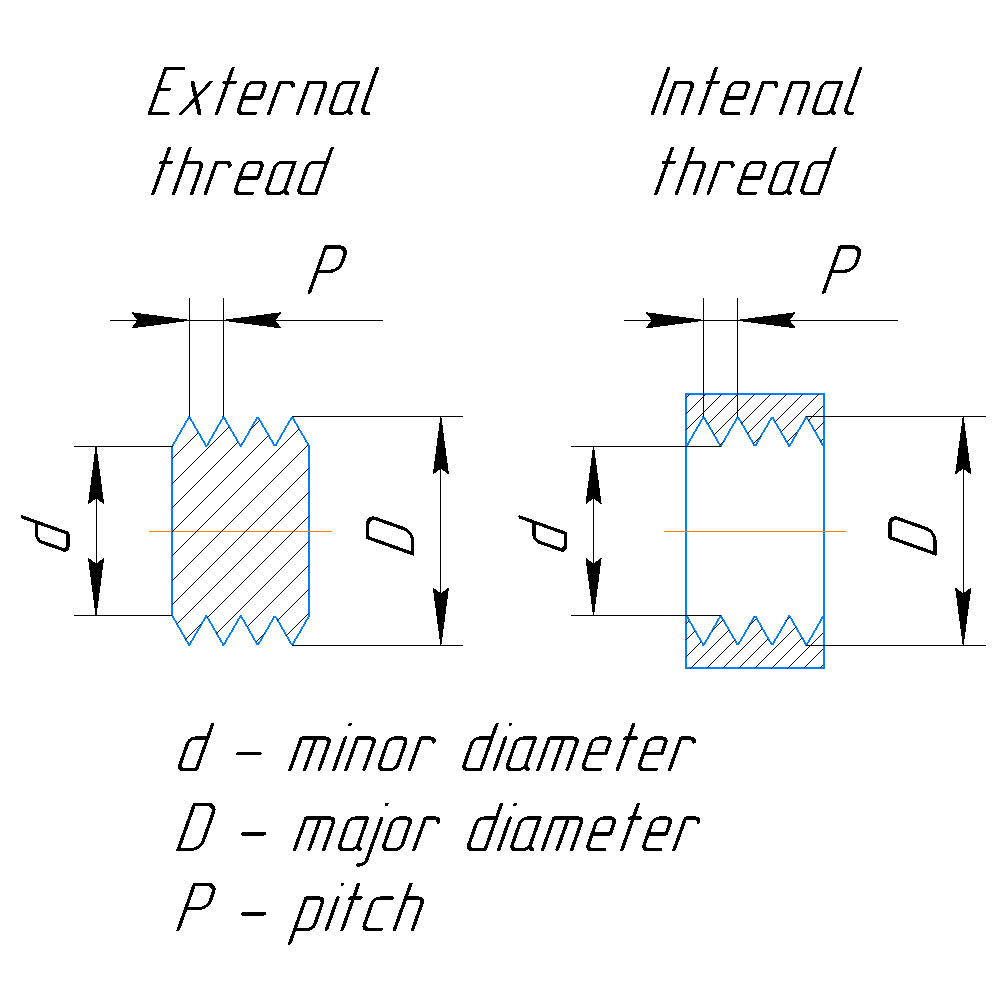Metric threads are described by capital M (stands for 'Metric') followed by major diameter (in millimeters), 'x' sign and thread pitch (in millimeters). So, if you see M52x0.75, you know this is metric thread with 52mm major diameter and 0.75mm pitch.

To measure a thread correctly, you should understand few basic things.

1. All metric threads are designated by major diameter.
2. Threads with same diameter may have different pitch. They are not compatible then.

So, if you need to determine external thread, you should just measure external diameter (D) and pitch (P).

If you need to determine internal thread, you should measure internal diameter (d), pitch (P), and add pitch to indernal diameter - this will give you approximate external diameter (D). d + P = D.

Best tool to measure diameters is caplier. Rulers are not precise enough.

Measuring pitch is more tricky. Best way is to use special thread gauge. They provide profile pattern for most common thread pitches (metric and Imperial). If don't have thread gauge, you can press a piece of paper to the thread and measure distance between imprints. You can achieve higher preciseness if you measure distance between first and last thread imprint, and divide it by number of spaces.

For example, you got imprints of 5 thread tops on paper. Distance between tops 1 and 5 is 3 mm. You should divide this number by 4 (quantity of spaces) - this will give you 0.75mm thread pitch.